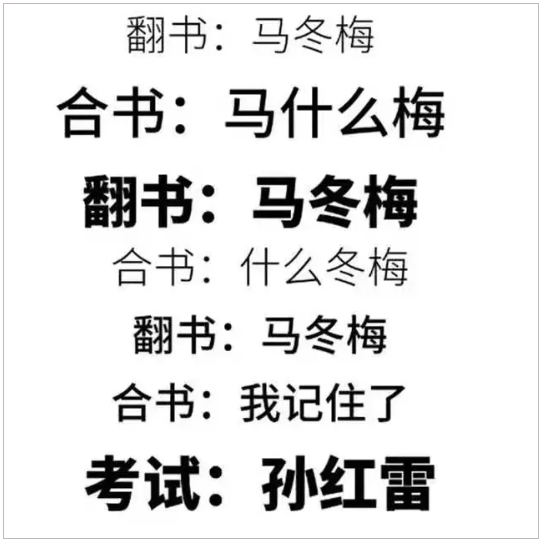# 一级造价师考试常用公式这么多，记了总忘该怎么办？1.预备费=基本预备费+涨价预备费

2.基本预备费=(工程费+工程建设其他费)×基本预备费率

3.工程费=设备及工器具购置费+建筑安装工程费用

4.价差预备费

5.静态投资=工程费+工程建设其他费+基本预备费

6.设备租赁的净现金流量=销售收入-经营成本-租赁费用-与销售相关的税金-所得税率×(销售收入-经营成本-租赁费用-与销售相关的税金)

7.建设期贷款利息=Σ(年初累计借款+本年新增借款÷2)×贷款利率

8.固定资产总投资=静态投资+涨价预备费+投资方向调节税+建设期贷款利息

9.生产性建设项目拟建项目总投资=固定资产总投资+流动资金

10.非生产性建设项目总投资=固定资产投资

11.建设项目总造价=固定资产投资总额

12.项目的流动资金=拟建项目固定资产总投资×固定资产投资流动资金率

13.实际利率=(1+名义利率÷年计息次数)年计息次数-1=(1+r÷m)m-1

14.流动资金=流动资产-流动负债

15.流动资产=应收(或预付)账款+现金+存货

16.流动负债=应付(或预付)账款

17.应收帐款=年销售收入÷年周转次数

18.现金=(年工资福利费+年其他费)÷年周转次数

19.存货=外购原材料、燃料+在产品+产成品

20.外购原材料、燃料=年外购原材料、燃料动力费÷年周转次数

21.在产品=(年工资福利费+年其他制造费+年外购原料燃料费+年修理费)÷年周转次数

22.产成品=年经营成本÷年周转次数

23.销售税金及附加=销售收入×销售税金及附加税率

24.利息I=总金额F-本金P

25.利率i=单位时间内所得的利息额÷本金P×100%

26.F=P(1+i)n

27.F=A[(1+i)n-1]÷i

28.P(1+i)n= A[(1+i)n-1]÷i

29.投资收益率R=年净收益(或年平均收益)÷投资总额×100%

30.总投资利润率Ra=(F+Y)÷K×100%

F---正常年销售利润;Y---正常年贷款利息;

K---总投资(建设投资+流动资金)

F=销售收入-经营成本-折旧费-摊销费-税金-利息

31.自有资金利润率Re=F÷Q×100%Q---自有资金

32.静态投资回收期Pt=K÷AK---总投资A---每年的净收益=(CI-CO)t

33.Pt=(累计净现金流量出现正数的年份数-1)+上一年累计净现金流量的绝对值÷出现正值年份的净现金流量

34.报考投资回收期Pt，=(累计净现金流量现值出现正数的年数-1)+上一年累计净现金流量现值的绝对值÷出现正值年份的净现金流量的现值

35.利息备付率(已获利息倍数)=税息前利润÷当期应付利息费用

36.税息前利润=利润总额+计入总成本费用的利息费用

37.当期应付利息=计入总成本费用的全部利息

38.偿债备付费=可用于还本付息的资金÷当期应还本付息金额

39.净现值率NPVR=NPV÷Kp Kp—投资现值

40.增量投资收益率R(2-1)=(C1-C2)÷(K2-K1)×100%

41.增量投资回收期Pt(2-1)=(K2-K1)÷(C1-C2)

42.增量投资回收期Pt(2-1)=(K2/Q2-K1/Q1)÷(C1/Q1-C2/Q2):业务量不同情况下。

43.综合费用法Sj=Kj+PcCj

44.年平均使用成本=(P-LN)÷N+设备的平均年度经营成本(P-LN)÷N----设备的平均年度资产消耗成本P----设备目前实际价值​LN---第N年末的设备净残值

45.经济寿命N0=[2(P-LN)÷λ]0.5

46.设备租赁的净现金流量=销售收入-经营成本-租赁费用-与销售相关的税金-所得税率×(销售收入-经营成本-租赁费用-与销售相关的税金)

47.租赁费用主要=租赁保证金占用损失+租金+担保费

48.附加率法：每期租金R=P(1+Ni)÷N+Pr=P(1÷N+i+r)P----租赁资产的价格N----总租期数i----与总租期数对应的折现率r----附加率

49.年金法分两种支付方式：期末等额支付R=P(A÷P，i,N)期初等额支付R= P(A÷P，i,N)÷(1+i)50.购买设备的净现金流量=销售收入-经营成本-设备购置费-贷款利息-与销售相关的税金-所得税率×(销售收入-经营成本-折旧-贷款利息-与销售相关税金)

50.购买设备的净现金流量=销售收入-经营成本-设备购置费-贷款利息-与销售相关的税金-所得税率×(销售收入-经营成本-折旧-贷款利息-与销售相关税金)

• #### 【工程计价】 关于项目投资估算的作用，下…